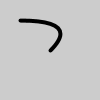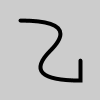Name

Examples```noFill()
strokeWeight(4)
beginShape()
vertex(20, 20)
endShape()
``````noFill()
strokeWeight(4)
beginShape()
vertex(20, 20)
vertex(80, 60)
endShape()
```
Description Specifies vertex coordinates for quadratic Bezier curves. Each call to quadraticVertex() defines the position of one control points and one anchor point of a Bezier curve, adding a new segment to a line or shape. The first time quadraticVertex() is used within a beginShape() call, it must be prefaced with a call to vertex() to set the first anchor point. This function must be used between beginShape() and endShape() and only when there is no MODE parameter specified to beginShape(). Using the 3D version requires rendering with P3D (see the Environment reference for more information).
Syntax
```quadraticVertex(cx, cy, x3, y3)
quadraticVertex(cx, cy, cz, x3, y3, z3)
```
Parameters
cx float: the x-coordinate of the control point float: the y-coordinate of the control point float: the x-coordinate of the anchor point float: the x-coordinate of the anchor point float: the y-coordinate of the anchor point float: the z-coordinate of the control point float: the z-coordinate of the anchor point
Related curveVertex()
vertex()
bezierVertex()
bezier()

Updated on Mon Sep 21 15:53:25 2020.

If you see any errors or have comments, please let us know.